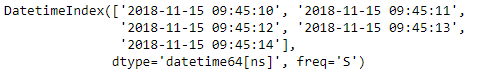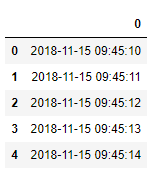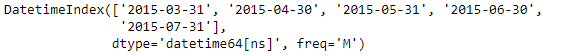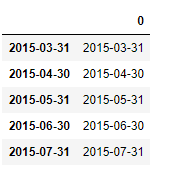Related Articles
Python | Pandas DatetimeIndex.to_frame()
• Last Updated : 29 Dec, 2018

Python is a great language for doing data analysis, primarily because of the fantastic ecosystem of data-centric python packages. Pandas is one of those packages and makes importing and analyzing data much easier.

Pandas` DatetimeIndex.to_frame()` function create a DataFrame with a column containing the Index. By default the labels of the DatetimeIndex object is used as an index for the newly constructed Dataframe.

Syntax: DatetimeIndex.to_frame(index=True)

Parameters :
index : Set the index of the returned DataFrame as the original Index

Return : DataFrame containing the original Index data.

Example #1: Use `DatetimeIndex.to_frame()` function to create a DataFrame object from the given DatetimeIndex object. Also set the index value to `False`

 `# importing pandas as pd``import` `pandas as pd`` ` `# Create the DatetimeIndex``# Here 'S' represents secondly frequency ``didx ``=` `pd.DatetimeIndex(start ``=``'2018-11-15 09:45:10'``, freq ``=``'S'``, periods ``=` `5``)`` ` `# Print the DatetimeIndex``print``(didx)`

Output :Now we want to construct a DataFrame out of the DatetimeIndex object.

 `# construct the DataFrame``didx.to_frame(index ``=` `False``)`

Output :As we can see in the output, the function has returned a DataFrame object constructed from the didx DatetimeIndex object.

Example #2: Use `DatetimeIndex.to_frame()` function to create a DataFrame object from the given DatetimeIndex object.

 `# importing pandas as pd``import` `pandas as pd`` ` `# Create the DatetimeIndex``# Here 'M' represents monthly frequency ``didx ``=` `pd.DatetimeIndex(start ``=``'2015-03-02'``, freq ``=``'M'``, periods ``=` `5``)`` ` `# Print the DatetimeIndex``print``(didx)`

Output :Now we want to construct a DataFrame out of the DatetimeIndex object.

 `# construct the DataFrame``didx.to_frame(index ``=` `True``)`

Output :As we can see in the output, the function has returned a DataFrame object constructed from the didx DatetimeIndex object.

Attention geek! Strengthen your foundations with the Python Programming Foundation Course and learn the basics.

To begin with, your interview preparations Enhance your Data Structures concepts with the Python DS Course.

My Personal Notes arrow_drop_up